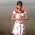## Thursday, July 28, 2011

### Logarithms in Psychology (Fitt's Law)

Logarithms can be applied to Psychology too! In the introduction of logarithms, we introduced 3 laws in Logarithms in Psychology. They are
1) Fitt's Law
2) Hick's Law
3) Weber-Fechner Law

Basically, these are laws used to calculate human reaction time. Fitt's Law is model the act of pointing, either physically touching an object with a hand or finger, or virtually, by pointing to an object on a computer monitor using a pointing device.

The person who invented this Law, Fitt's, discovered a formal relationship that models speed or accuracy tradeoffs in rapid, aimed movements. According to Fitt's Law, the time to move and point to a target of width W at a distance A is a logarithmic function of the spatial relative error.

There are a few variations to this magical Fitt's Law. (the traditional way and the improved method) However, they all use the concept of logarithms. The variations are the use of different symbols. However, if you closely compare the different formulas, they use the same theory and are the same.

Here's the formula that we find is the easiest to understand:Definitions:
T -- Average time taken to complete the movement
a -- Represents the start and stop time of the device (intercept)
b -- Represents the inherent speed of the device (slope)
*Note that a and b can be determined by fitting a straight line to the measured data
D -- The distance from the starting point to the center of the target
W -- The width of the target measured along the axis of motion.

However, traditionally, researchers used different symbols for this formula.
T can be replaced by MT, which is the movement time
1 can be replaced c, which is a constant of 0, 0.5 or 1. (We use 1 as c)
D can be replaced by A, which is the amplitude
And W corresponds to "accuracy"

By using this formula, we can predict speed of the action of pointing to or tapping an object.http://cdn.sixrevisions.com/0128-03_diagram.jpg

As you can see from this diagram, the object's distance and width does play a very important role in calculating the speed and accuracy of the action.

#### 1 comment:

1.thank you very much!!! that was really a help for me